# Chapter 7: Matrices and Determinants - Online Test

Q1. If aij = 1/2 (3i − 2j ) and A = [a ij] 2×2 is
Explaination / Solution: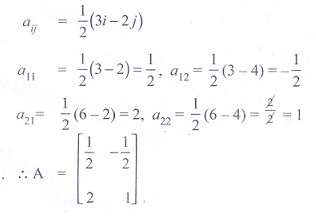Q2. What must be the matrix X, if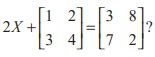Explaination / Solution:Q3. Which one of the following is not true about the matrixExplaination / Solution:
No Explaination.

Q4. If A and B are two matrices such that A + B and AB are both defined, then

Explaination / Solution: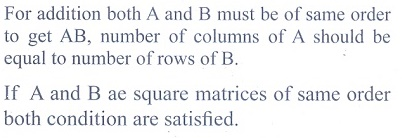Q5. If A =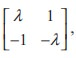then for what value of λ, A2 = O ?
Explaination / Solution:Q6. If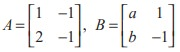and ( A + B )2 = A2 + B2 then the values of a and b are
Explaination / Solution:Q7. If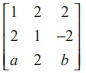is a matrix satisfying the equation  AAT = 9I, where I is 3 × 3 identity matrix, then the ordered pair (a, b) is equal to
Explaination / Solution: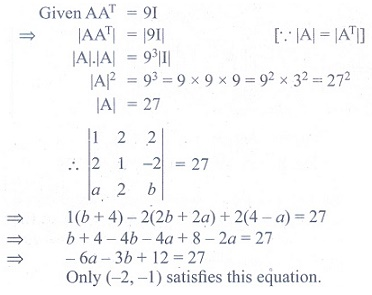Q8. If A is a square matrix, then which of the following is not symmetric?
Explaination / Solution:Q9. If A and B are symmetric matrices of order n, where ( A ≠ B), then
Explaination / Solution:Q10. If Aand if xy = 1, then det ( AAT ) is equal to
Explaination / Solution: